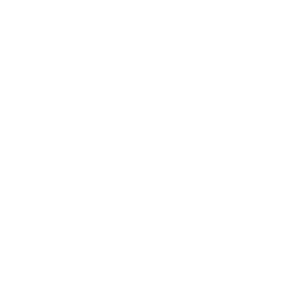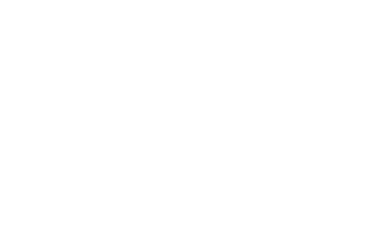# Mathematics Mocks Revision 21/09/2016

1. A sum of money is divided between three people, Tom, Mary and Lucy in the ratio 5:3:1. If  Mary has sh.700 more than Lucy, calculate how much Tom has.

2. In the figure below, O is the centre of the circle and OB bisects angel ABC. Given that <BAC= 40 deg, find <ABO3. Find the integral values of y of which satisfy the inequalities below.

5 ≤ 3y + 2 and 3y –14 ≤ -2

4. The position vector of points A and B relative to the origin O are a and b respectively. Given that a = 4i + 3and b = i + 2j, find the modulus of the vector a + b

5. Tap A takes one hour to fill a tank with water. If tap B takes y hours to remove the same water and both taps takes 1 ½ hours to fill the tank, find the value of y.

6. Find the length QR of the following triangle if PR=3.7cm PQ=4cm and <PQR = 63 degThe questions above were discussed in our study group.

• ✔ To read offline at any time.
• ✔ To Print at your convenience
• ✔ Share Easily with Friends / Students